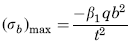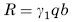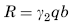Related Resources: calculators

### Simply Supported Uniform Loading over 2/3 of plate from fixed edge Stress and Deflection Equation and C

Flat Rectangular Plate, Three Edges Fixed, One Edge (a) Simply Supported Uniform Loading over 2/3 of plate from fixed edge Stress and Deflection Equation and Calculator.

Per. Roarks Formulas for Stress and Strain for flat plates with straight boundaries and constant thickness

Flat Rectangular plate, Three Edges Fixed, One Edge Uniform Loading over 2/3 of Plate

 Rectangular plate; Three Edges FixedUniform Loading over 2/3 of plate from fixed edgeAt x = 0, z = 0
StressReaction ForceStress at x = 0, z = 0.6bandAt x = 0, z = b
Reaction ForceAt x = ± a/2, z = 0.4b
StressReaction ForceWhere used:
E = Modulus of Elasticity (lbs/in2)
q = Total load or force to Plate (lbs/in2)
v = Poisson’s ratio (assumed to be 0.3)
t = plate thickness, (in)
a = plate length, (in)
b = plate width, (in)
σb = stress, (lbs/in2)
R = Reaction Force (lbs/in)
β1,2,3,4 = Constant From Table A
γ1,2,3 = Constant From Table A

Table A

 a/b 0.25 0.5 0.75 1 1.5 2 3 β1 0.02 0.08 0.164 0.274 0.445 0.525 0.566 β2 0.003 0.016 0.044 0.093 0.193 0.252 0.286 β3 0.012 0.043 0.081 0.108 0.112 0.091 0.066 β4 0.031 0.111 0.197 0.255 0.284 0.263 0.204 γ1 0.115 0.23 0.334 0.423 0.517 0.542 0.543 γ2 0.002 0.015 0.048 0.088 0.132 0.139 0.131 γ3 0.125 0.25 0.345 0.396 0.422 0.417 0.405

Reference:

Roarks Formulas for Stress and Strain, 7th Edition